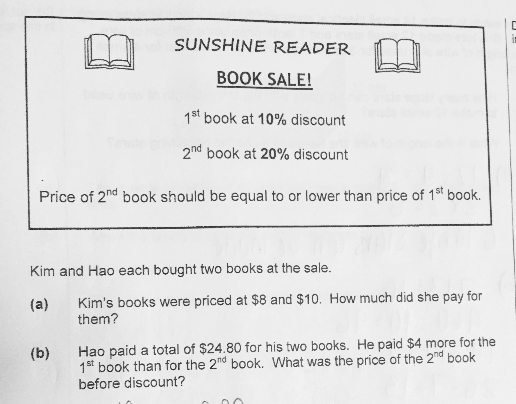# QuestionAnyone help me with attached question

Thanks

(a)
1st book ——- 10 x (100% – 10%) = 9.00
2nd book ——- 8 x (100% – 20%) = 6.40
6.40 + 9.00 = 15.40

(b)
24.80 – 4 = 20.80
20.80/2 = 10.40 (price of 2nd book after discount)
100% – 20% = 80% ——- 10.40
1% ——- 10.40/80 = 0.13
100% ——- 100 x 0.13 = 13

Ans : (a) \$15.40; (b) \$13.

0 Replies 1 Like ✔Accepted Answer

Finding answers to these questions is not easy you have to research and watch the different guides about it. The thesis formatting service shares some quick tips through videos that will help you out.

0 Replies 0 Likes

A) 1st book is \$10.

10% off 1st book = 0.9 x \$10 = \$9

20% off  2nd book = 0.8 x 8 = \$6.40

Total cost of book = 9 + 6.40= \$15.40#

B)

Let the cost of the lower priced book be = u

u + (u+4) = \$24.80

2u  + 4 = \$24.80

2u = 20.80

u = \$10.40

Before discount= \$10.40/0.8 = \$13#

0 Replies 2 Likes